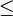### Thresholding (Iterative Method)

Thresholding is inevitably one of the mostly used image processing algorithm. It is told as the simplest method of image segmentation.

In this post, I want to introduce one thresholding algorithm that is powerful enough yet simple enough to implement. The method called "Iterative Method." Below is the step by step used in the algorithm:

1. An initial threshold (T) is chosen, this can be done randomly or according to any other method desired.
2. The image is segmented into object and background pixels as described above, creating two sets:
1. G1 = {f(m,n):f(m,n)>T} (object pixels)
2. G2 = {f(m,n):f(m,n)$\le$T} (background pixels) (note, f(m,n) is the value of the pixel located in the mth column, nth row)
3. The average of each set is computed.
1. m1 = average value of G1
2. m2 = average value of G2
4. A new threshold is created that is the average of m1 and m2
1. T’ = (m1 + m2)/2
5. Go back to step two, now using the new threshold computed in step four, keep repeating until the new threshold matches the one before it (i.e. until convergence has been reached).

Posted below is the Java source code I use to implement the algorithm.
```/*
Method Name: _calculateThreshold
Params     : image: BufferedImage -> Image representation in Grayscale colour space
Return     : double -> The computed threshold value
*/
private double _calculateThreshold ( BufferedImage image )
{
double totalThreshold = 0;
double averageThreshold = 0;

/* Get the image width and height */
int width = image.getWidth ();
int height = image.getHeight ();
```
```    /* Get the image data */
Raster data = image.getData ();
double[] pixel = new double[ width * height * 3 ];

data.getPixels ( 0 , 0 , width , height , pixel );

/*
Calculate threshold value as initial threshold by computing average threshold value
*/
for ( int row = 0 ; row < height ; row ++ )
for ( int col = 0 ; col < width ; col ++ )
{
int index = col * 3 + row * width * 3;
double gray = ( 0.3 * pixel[index] + 0.59 * pixel[index + 1] + 0.11 * pixel[index + 2] );

totalThreshold += gray;
}

averageThreshold = totalThreshold / ( double ) ( width * height );

/* Begin the iterative method */
while ( true )
{
float g1 = 0;
float g2 = 0;
int g1Count = 0;
int g2Count = 0;

for ( int row = 0 ; row < height ; row ++ )
{
for ( int col = 0 ; col < width ; col ++ )
{
int index = col * 3 + row * width * 3;
double gray = ( 0.3 * pixel[index] + 0.59 * pixel[index + 1] + 0.11 * pixel[index + 2] );

if ( gray > averageThreshold )
{
g1 += gray;
g1Count ++;
}
else
{
g2 += gray;
g2Count ++;
}
}
}

double m1 = g1 / ( double ) g1Count;
double m2 = g2 / ( double ) g2Count;
double m = ( m1 + m2 ) / 2;

if ( averageThreshold == m )
break;
else
averageThreshold = m;
}

/* Return the computed threshold value */
return averageThreshold;
}
```
Method below shows how we use the computed threshold value to convert our grayscale image to B/W (or so called binary image).
```/*
Method Name: _convertToBW
Params     : image: BufferedImage -> Coloured image representation
Return     : BufferedImage -> The B/W image
*/
private BufferedImage _convertToBW ( BufferedImage image )
{
/* Create a buffer to store the result */
BufferedImage result = new BufferedImage ( image.getWidth () , image.getHeight () , BufferedImage.TYPE_INT_RGB );

/* Get the image data */
int width = image.getWidth ();
int height = image.getHeight ();

/* Convert the image to grayscale */
ColorConvertOp op = new ColorConvertOp ( ColorSpace.getInstance ( ColorSpace.CS_GRAY ) , null );
op.filter ( image , result );

/* Calculate the average threshold */
double averageThreshold = this._calculateThreshold ( result );

/* Convert the image to B/W colour space */
WritableRaster data = result.getRaster ();
double[] pixel = new double[ width * height * 3 ];

data.getPixels ( 0 , 0 , width , height , pixel );

for ( int row = 0 ; row < height ; row ++ )
{
for ( int col = 0 ; col < width ; col ++ )
{
int index = col * 3 + row * width * 3;
double gray = ( 0.3 * pixel[index] + 0.59 * pixel[index + 1] + 0.11 * pixel[index + 2] );

/*
If the intensity is less than the given threshold, then make it black.
Otherwise, make it white
*/
if ( gray < averageThreshold )
data.setPixel ( col , row , new int[]
{
0 , 0 , 0
} );
else
data.setPixel ( col , row , new int[]
{
255 , 255 , 255
} );
}
}

/* Return the result */
return result;
}
```
Hope you find this post useful.